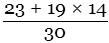# Easter algorithms from Nature, 1876

From Nature, 17 February 1876

{315}

A supposed error in the determination of the date when Easter Sunday should fall in the present year has been made the subject of communications to various metropolitan and provincial journals. We shall enter more fully into this question next week; meanwhile it may be stated that the presumed error is an imaginary one, according to the strict methods for ascertaining the date of Easter Sunday, which is correctly fixed by our almanacs to the 16th of April.

From Nature, 24 February 1876

{326}

WE revert to this subject with the view to reproduce the arithmetical rule to find Easter Sunday in the Gregorian Calendar, which was first given by the {327} eminent mathematician and astronomer Gauss, in Zach’s Monatliche Correspondenz, 1800.

 1. From 1800 to 1899 put m = 23, n = 4. „ 1900 to 2099 „ m = 24, n = 5.
 2. Divide the given year by 19, and call the remainder a. 3. „    „ by 4, „    „ b. 4. „    „ by 7, „    „ c. 5. Add m to 19 times a, divide the sum by 30, and call the remainder d. 6. Add together n, twice b, four times c, and six times d, divide the sum by 7, and call the remainder e.

Then Easter Sunday is March 22 + d + e, or d + e – 9 of April.
To apply this rule to the present year, we have—

 1 m = 23   n = 4 2 Forremainder is 14 a. 3 Forremainder is 0 b. 4 Forremainder is 0 c. 5 Forremainder is 19 d. 6 Forremainder is 6 e.

And Easter Sunday is March 22 + 19 + 6 = March 47 or April 16; or 19 + 6 – 9 of April = April 16.

Note.—The following are two exceptions to the above rule:—
1. If Easter Sunday is brought out April 26, we must take April 19.
2. If Easter Sunday results on April 25 by the rule, the 18th must be substituted when the given year, increased by one, and then divided by 19, leaves a remainder greater than 11.

From Nature, 20 April 1876

{487}

TO  FIND  EASTER

A NEW York correspondent sends us the following rule, which he states to be devised by himself, to find the date of Easter Sunday, perpetually:—

 To find Easter “for ever.” Divide By And call the Quotient Remainder The year of our Lord 19 — a „         „ 100 b c b 4 d e b + 8 25 f — b – f + 1 3 g – 19a + b – d – g + 15 30 – h c 4 i k 32 + 2e + 2i – h – k 7 – l a + 11h + 22l 451 m – h + l – 7m + 114 31 n o

n is the number of the month of the year and o + 1 is the number of the day of the month on which Easter falls.